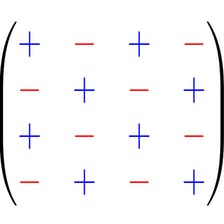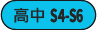# Matrix Algebra for Engineers

HKUST
Enrollment is ClosedThis course is all about matrices, and concisely covers the linear algebra that an engineer should know. The mathematics in this course is presented at the level of an advanced high school student, but typically students should take this course after completing a university-level single variable calculus course. There are no derivatives or integrals in this course, but students are expected to have attained a sufficient level of mathematical maturity. Nevertheless, anyone who wants to learn the basics of matrix algebra is welcome to join.

The course contains 38 short lecture videos, with a few problems to solve after each lecture. And after each substantial topic, there is a short practice quiz. Solutions to the problems and practice quizzes can be found in instructor-provided lecture notes. There are a total of four weeks in the course, and at the end of each week there is an assessed quiz.

#### Modules

###### 1. Matrices

Matrices are rectangular arrays of numbers or other mathematical objects. We define matrices and how to add and multiply them, discuss some special matrices such as the identity and zero matrix, learn about transposes and inverses, and define orthogonal and permutation matrices.

###### 2. System of Linear Equations

A system of linear equations can be written in matrix form, and can be solved using Gaussian elimination. We learn how to bring a matrix to reduced row echelon form, and how this can be used to compute a matrix inverse. We learn how to find the LU decomposition of a matrix, and how to use this decomposition to efficiently solve a system of linear equations with evolving right-hand sides.

###### 3. Vector Space

A vector space consists of a set of vectors and a set of scalars that is closed under vector addition and scalar multiplication and that satisfies the usual rules of arithmetic. We learn some of the vocabulary and phrases of linear algebra, such as linear independence, span, basis and dimension. We learn about the four fundamental subspaces of a matrix, the Gram-Schmidt process, orthogonal projection, and the matrix formulation of the least-squares problem of drawing a straight line to fit noisy data.

###### 4. Eigenvalues and Eigenvectors

An eigenvector of a matrix is a nonzero column vector that when multiplied by the matrix is only multiplied by a scalar, called the eigenvalue. We learn about the eigenvalue problem and how to use determinants to find the eigenvalues of a matrix. We learn how to compute determinants using the Laplace expansion, the Leibniz formula, or by row or column elimination. We also learn how to diagonalize a matrix using its eigenvalues and eigenvectors, and how this leads to an easy calculation of a matrix raised to a power.

## Course Staff### Jeffrey R. Chasnov

Jeffrey R. Chasnov is a Professor of Mathematics at the Hong Kong University of Science and Technology, where he has been teaching since 1993. He is an expatriate American from New York and California, and earned his BA from UC Berkeley and PhD from Columbia University, with post-doctoral fellowships at NASA, Stanford, and Grenoble, France, and a sabbatical semester at Harvey Mudd College. He is the author of numerous research articles in fluid turbulence and mathematical biology, and has authored online textbooks and videos for his courses on differential equations, matrix algebra, mathematical biology and scientific computation. Before and after work, he enjoys family life, swimming and tennis, and takes great pleasure in his family’s annual camping and skiing vacations.

1. Course Number

matrix-algebra
2. Classes Start

3. Classes End